�7#P�_�O�^^^^^^(�^�^�^�^� ^�"^�^�B_x^�_� _�_�*_�^_�_� _�_�_�_�_�_�_�_�_� Graphs and Curve Fitting

# APPENDIX C

## Graphs and Curve Fitting

Experimental work in science frequently involves studying the relationship between two interacting variables. One example would be how the velocity of a falling body varies with time. In such an experiment, the dependent variable (velocity in the above example) is measured at a series of values of the independent variable (time in the example). Data from such an experiment might be recorded as in the tabular form below.
 Velocity in Free Fall t (sec) v (m/sec) 0 15.0 +/- 2.3 1 24.8 +/- 2.2 2 34.1 +/- 2.1 3 44.4 +/- 2.5 4 55.0 +/- 2.9 5 64.0 +/- 3.2

Table 1

Numbers in a table like this do not convey the relationship (or lack thereof) between the variables to the human mind very easily. This can be done with more immediacy by drawing a graph.

## A. Drawing Graphs

A (2-dimensional) graph is a picture in which a relationship between two variables is expressed as a line drawn in a plane.

Any point in a plane can be located by reference to two perpendicular rays, called axes, given in that plane. Consider the segment from the intersection of the axes (called the origin) to the point in question. By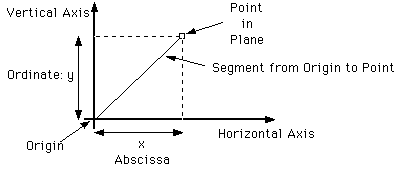Figure 1

measuring off the perpendicular projections of this segment onto the axes the point is uniquely located. And, conversely, these projections, called coordinates, are uniquely determined by the point. The distance along the vertical axis is called the ordinate. Because the ordinate is often denoted by "y" it is commonly called the y-coordinate and the vertical axis is commonly called the y-axis. The distance measured along the horizontal axis is called the abscissa; since it is often denoted by "x" it is commonly called the x-coordinate and the horizontal axis is commonly called the x-axis.

In this way a point relates two numbers, its coordinates. And thus a line in the plane determines a relationship between two variables; namely the relationship between the abscissa and ordinate of all points on the line.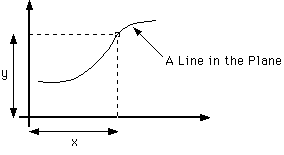Figure 2

If a few of the corresponding values of the independent and dependent variables of a relationship are known they can be plotted on a graph. (It is conventional to plot the independent variable on the horizontal axis and the dependent variable on the vertical axis.) That is, for the related variables, a point is found, and marked, by measuring a distance on the horizontal axis proportional to the independent variable and a distance on the vertical axis proportional to the dependent variable. Then, there is a one-to-one correspondence between the plotted points and the related values of the variables. These points, presumably, are part of the line which expresses the relationship between the variables.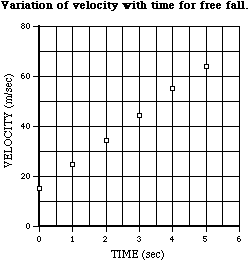Figure 3

For instance, the values of the previous example are plotted above. From this picture the human eye can clearly see the regular relationship that exists between the variables in a direct way that is not so readily apparent from looking at the table of numbers to which it corresponds.

If a smooth curve is drawn through such a plot of points it may give some idea of the curve that expresses the functional relationship between the variables. The more points, of course, the more detail and confidence there will be. In this way, intermediate values (which have not actually been measured) may be estimated by interpolating between the measured points with a smooth curve. Also, drawing graphs like this is a useful way to learn about and estimate the functional form of a relationship between measured variables.

In the case of the example, it can be seen (on next page) that a straight line fits rather well, with the plotted points deviating only slightly from it. The equation of such a straight line is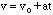(1)

for some constants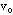and a. These constants can be read right off the graph. The intercept (where the graph intercepts the y-axis), 15m/s, isand the slope, about 10m/s2, is a. In this way an algebraic expression estimating the relationship between the variables in the table can be obtained.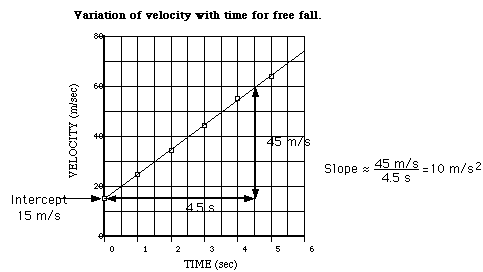Figure 4

Note that in the above plot it is not necessary to use a particular scale relating the variables to the actual distances on the axes. As long as the distances on the axes are proportional to the variables the points will fall near a straight line. And as long as the axes are marked off in terms of the variables we can read the value of the variable off directly and don't even have to worry about what the scale actually is. The scale can be selected purely for convenience. A variety of patterns of graph paper can be purchased for making such plots. Or computer programs (such a Cricket Graph) can be used.

## B. Indicating Errors

Any plot of experimental data must include an indication of the uncertainty. Errors are indicated on a plot by error bars above and below the plotted point which show the range of possible values.

The example has been replotted, above, to include error bars. Note that when the bars are included it is apparent that the line drawn through the points could be just as well be moved up or down or changed in slope a bit and still pass inside the error bars. This is an indication of the uncertainty of the line drawn through the points. In this way, the uncertainty in the parameters of the straight line fit obtained above (intercept and slope) could be estimated.

## C. Transformation of Variables

Sometimes the relationship between data variables may not be linear but by a simple transformation can be converted to a linear form. For instance, if the pressure of a sample of gas were measured as a function of volume it should obey,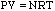, (2)

the Ideal Gas Law. If this were plotted it would yield the hyperbola of Boyle's Law but it would not be readily apparent to the eye how accurately the plotted curve did so. However,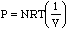, (3)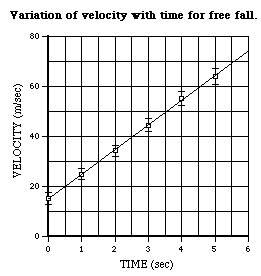Figure 5

is a linear relationship. If P is plotted against the reciprocal of V it should yield a verifiable straight line. A test of the law would be that the intercept should be zero. And a value for NRT could be gotten from the slope.

## D. Logarithmic Graphs

For some non-linear relationships, one can use similar techniques involving logarithms. For instance, if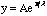or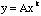(4)

then taking logarithms (log denotes the logarithm to the base 10) of these equations yields,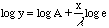and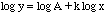, (5)

linear relationships which can be treated as before.

 Free Fall Distance vs. Time Thermal Conductivity time (sec) distance (m) time (min) DT (deg.C) 0.0 0.00 0 23.2 0.5 1.29 1 21.1 1.0 4.9 2 19.5 1.5 11.0 3 18.0 2.0 19.6 4 16.4 2.5 30.6 5 15.0 3.0 44.0 6 13.8 3.5 60.0 7 12.7 4.0 78.4 8 11.9 4.5 99.2 9 11.0 5.0 122.5 10 10.4

Table 2

To illustrate these logarithmic techniques, consider the following sets of data. The first table is for free fall and the second table is for cooling due to thermal conductivity. If rectangular plots are made of these data the results clearly fall near reasonably smooth curves but the nature of these curves is not apparent by inspection. Use of logarithmic plots is much more informative.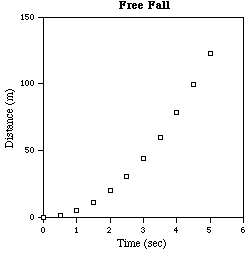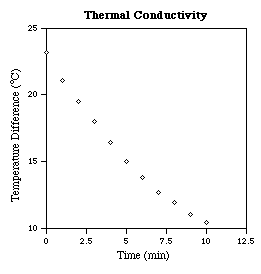Figure 6

In the case of exponential behavior, if the logarithm of the dependent variable is plotted against the independent variable a straight line results. The intercept determines the proportionality constant and the slope, .43/l (log e ~ .43), determines the decay constant. Since, in this plot, a logarithm is taken of only one of the variables (the dependent one) this is called a semi-log plot. Graph paper is available in which the y-axis is marked off with a logarithmic scale so that such a plot can be make directly without having to actually calculate any of the logarithms. On such paper, the value of l can be read off directly as .43 times the change of the independent variable required for the dependent variable to change by a decade. For Example,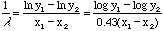. (6)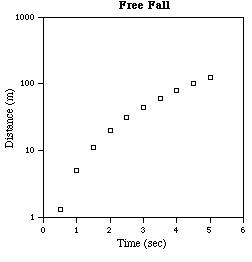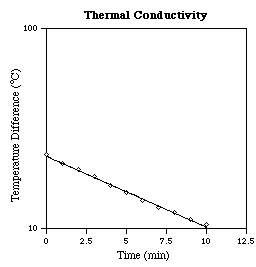Figure 7

Making log-log plots of the example data reveals that the thermal conductivity data clearly is not a power law. However, the free fall data falls rather well on a straight line, indicating something very near a power law.

Observe that in one decade on the x-axis (say from 1 to 10) the line rises about two decades on the y-axis; so the slope is about two, representing a quadratic power law. Also for x = 1 the y value is 4.9, the proportionality constant. Thus, examination of the graph determines the relation between the variables to be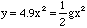(7)

where g = 9.8 (the magnitude of the acceleration due to gravity in the units used).

Making a semi-log or a log-log plot is particularly useful in determining if, and the extent to which, the variables in a set of data are related exponentially or as a power. And the functional parameters of such relationships can be estimated from the graphs.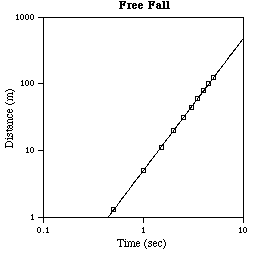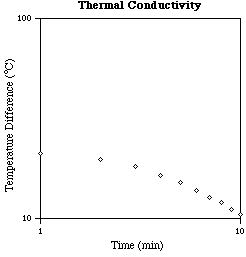Figure 8

## E. Other Types of Plots

There are other types of plots that are convenient when dealing with certain types of data.

For instance, when the independent variable is an angle it would be appropriate to plot the dependent variable versus an actual angle on a polar plot. An example of this would be the angular distribution of radiant intensity transmitted through a small aperture. Special polar graph paper is available for such purposes.

In addition, it may be useful to use axes with logarithmic scales even if producing a straight line (as in those cases considered above) is not an object. For instance, if a wide range values of a variable (several factors of ten) needs to be plotted this allows the small values to be displayed legibly rather than squashed up together near zero. Some physical phenomena (like the sensation of intensity in the human ear) are logarithmic in nature and so most naturally displayed with a logarithmic scale. Logarithmic scales also facilitate identifying features related by ratios since equal distances on a logarithmic scale represent the same factor.

## F. Least Squares Fitting

Drawing a smooth curve or a straight line on a graph is a useful but rather subjective procedure. These kinds of estimates can be made in a more precise and systematic way by what is called the method of least squares.

Only the case investigating the extent of a linear dependence between y and x will be considered here. That is, a function of the form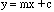(8)

which fits a set of data will be sought. (This same sort of process may be used, as above, for exponential and power law behavior by taking logarithms.) The problem consists of finding the slope m and intercept c,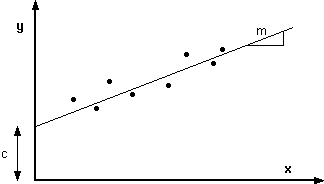Figure 9

which best fits given data. If there are n pairs of measurements,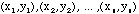the object is to obtain the "best" fit so that,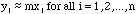as closely as possible.

To do this the idea is to form some measure of how closely a line fits the data and find the values of the slope and intercept which optimize this measure. The sum of the squares of the deviations,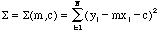, (9)

is a quantity which increases the further any of the data points lie from the line and gives more weight to points farther from the line. There is nothing profound or magic about the use of this quantity; it is merely a plausible, but ad hoc, measure of the fit. The line whose values of m and c minimize the above quantity is a plausible one to use as a best fit. This is the method of least squares.

The quantity S(M, c) will be a minimum when both partial derivatives vanish,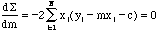and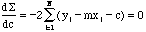(10)

These two equations can be rewritten as a pair of linear equations for the parameters of the fit, m and c,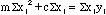and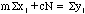. (11)

Thus, the best straight line goes through the point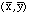where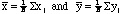. (12)

In terms of these quantities we can solve for the parameters of the fit,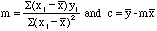. (13)

There are many pocket calculators which can calculate the necessary sums automatically. And there are many computer programs available with facilities for doing least squares fitting.

Send comments, questions and/or suggestions via email to wolfs@nsrl.rochester.edu.
u}%�Pilot's Folder�%�Pilot's Folder� ���b8P�P�� @ <IYch��4h����*x� W����Yx~�����&.Zy�����\$,Xw�����"*Vu����� 5 � � � � � � < t �  N � � �  a � � I � �'u� Yt�j����������������������������������������������������������������������������������������������������cS��/V���f��E��/{�]���+a��7l�k���5�� k� CDO��&t�[��� HIM�� 5 > D � � �!!f!�!�!�""c"~"�"�#-#y#�#�#�#�#�\$*\$.\$7\$�\$�\$�% ���������������������������������������������������������������������������������������������������c% %Z%u%~%�%�&&-&b&f&u&�&�&�&�''('M'Z'd'�'�'�'�(( ((:(\(b(l(�(�(�(�(�) ))>)])g)�)�)�)�** *3*R*\*~*�*�*�*�++'+F+P+r+�+�+�+�+�,,;,E,g,�,�,�,�,�--0-:-\-�-�-�-�-�..%./.Q.}.�.�.�.�.�//\$/F/r/�/�/����������������������������������������������������������������������������������������������������c/�/�/�000;0g0�0�0�0�0�11101\1}1�1�1�1�1�22%2Q2r2|2�2�2�2�2�33>3P3[3�3�4@4�4�4�5�5�5�6D6�6�7*7x7�88]8�8�8�99O9[::":-:x:�;;;d;�;�<>o? ?\$?.?3?g?h?s?�?�@+@y@�AA'AqA�B BXB����������������������������������������������������������������������������������������������������cB�B�C=C�C�C�C�C�C�DJD�D�D�E:E�E�E�FFcF�F�GGRGmG�G�HHGHPH�H�I IXI�I�I�JJOJ�J�K9K�K�K�L8LBLrL�L�L�MMMM]M�M�M�N)N-N3N|N�N�N�N�OGOnOrO�P P.P2P7P�P�P�P����������������������������������������������������������������������������\$� K �O�P��&M+�1|<�G�O��� ��N���� ��"��#��D��PP�� P�)% /�B�P�*+,-. !"����"�((�(�)343�8�9~�HH�@����Xe(��HH� @�'����X=�/��`����B@H -:StyleWriter Times�O�O���O�E� �